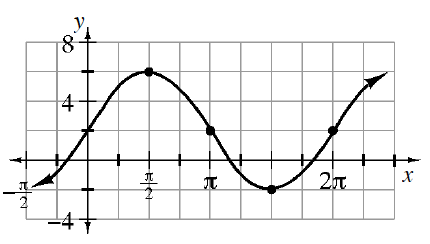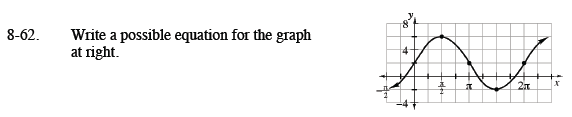Home > CCA2 > Chapter 8 > Lesson 8.1.3 > Problem8-62

8-62.

Write a possible equation for the graph below. Homework Help ✎Determine how the graph is altered from the graph of y = sin(x). What is the amplitude? Where is the midline?

y = 2 + 4 · sin(x)